Office Applications and Entertaiment, Magic SquaresForm5 About the Author

Pan Magic Squares 5 x 5, Interactive Solution, Sudoku Method

Introduction

Pan Magic Squares of the 5th order can be constructed by means of following Sudoku Comparable Method:

1. Fill the first row of square A with the numbers 0, 1, 2, 3 and 4.
While starting with 0 there are 4! = 24 possible combinations;
2. Copy the first row of square A into the following rows of square A, however every time two places to the left.
The numbers 0, 1, 2, 3 and 4 will occur now only one time in every row, column or (pan) diagonal;
3. Fill the first row of square B with the numbers 0, 1, 2, 3 and 4.
While starting with 0, 1 there are 3! = 6 possible combinations;
4. Copy the first row of square B into the following rows of square B, however every time two places to the right.
5. Construct the final square C by means of the matrix operation C = 5 * A + B + .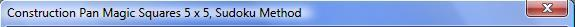Squares

A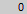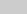B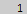CC = 5 * A + B + 

First Row A

 1 2 3 4 1 2 3 4 1 2 3 4 1 2 3 4

First Row B

 2 3 4 2 3 4 2 3 4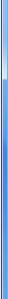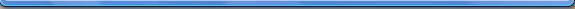Procedure:

1. Select the first row of matrix A with the left  selection buttons, and confirm by pushing button 'Matrix A'.

2. Select the first row of matrix B with the right selection buttons, and confirm by pushing button 'Matrix B'.

3. Press the button ‘Show Square’ to calculate and visualise the resulting Pan Magic Square.

4. Press the button ‘Show Class’ to visualise the related Class Cn based on shifting, rotation and reflection of Pan Magic Square C.

The possible combinations of square A and B described above will result in 24 * 6 = 144 solutions, which are shown in Attachment 5.3.4.

Each of these 144 Pan Magic Squares will result in a unique Class Cn and finally in the 200 * 144 = 28800 possible Pan Magic Squares of the 5th order as shown in Attachment 5.2.6.

Have Fun!About the Author